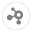44
Views
4
Solved
[Formula] Formula / Flee Question
QuestionForge component by Caio Santana Magalhães
Application Type

Hello everyone,

So i am using Formula forge asset which uses the .NET flee expression evaluator. It's working great but i have some custom specification that i don't know i can achieve using this.

Basicly i am building like a custom exceptions builder for the clients, so they can use fields of a form as variables and then i replace the variables with the real time values  and evaluate the expression to check if it's either True or False. If it's True then i will highlight the fields.

To give a specific example:

1. i have a form with, for example, 3 input fields, each input has a code, let's say Code_1, Code_2, Code_3

2. my formula string will be like this: Code_1 = "sometest" Or Code_2 = "tbd" Or Code_3 = "123"

Real time values: Code_1 = "outsystems", Code_2 = "tbd", Code_3 = ""

3. After evaluating this with Flee if any of this fields gets a true result, all 3 fields will be highlighted red, but i would like to highlight only the ones that really return true, in that example only the Code_2 field.

Any ideas if that possible?

Thank youCaio Santana Magalhães

MVP
Solution

Hi @Ruben Magalhães, sorry that I missed this question.

You probably already found another way around that ever since you posted this question. If you did, would you mind sharing the solution that you found?

As for your question, I would first consider trying to find a way of not using Formula/Flee for this, since I believe that using this component for this use case might add too much complexity.

If your form inputs are completely dynamic, you could try parsing those variables onto a list and running a For Each on that list, checking if their values match the expected values, and setting a Boolean flag on the current record indicating that this particular form field should be highlighted on the UI.

Now, if you absolutely still want to use Formula/Flee for this, there are ways of doing this using the FormulaEvaluateText Action. One idea is to return a string with the field names that successfully matched your expected values as a CSV. See the following formula as an example:

```If(Code_1 = "sometest", "Code_1", "") + "," +
If(Code_2 = "tbd", "Code_2", "") + "," +
If(Code_3 = "123", "Code_3", "")```

With the resulting CSV, you should be able to use String_Split on it and create logic for highlighting the form inputs that were found in the CSV.Again, I believe there are simpler ways of achieving this without using Formula/Flee, but anything is possible.Ruben Magalhães

Hello @Caio Santana Magalhães ,

Actualy i didn't found a solution for that, and the client discarted the idea of highlighting the fields (at least for now). Plus i will have very complex expression with custom functions aswell and the expression can be in many different formats.
I am still using formula, however just for the final part of evaluating the expression, all functions and variables i replace in outsystems itself.Maykell Ribeiro

I'm trying to calculate a formula using the value 10^(1/2), but the component is not considering the value of 10^(1/2) which would be 3.16227766and yes 1.Could anyone tell me how I should put together this formula?For example: 10*10^(1/2), should return 31.6227766 and using the component is returning 10.Maykell Ribeiro

I managed to find 2 solutions to the above problem.We can use the floor() function on the first number of the division, which would be the smallest, or write it as a decimal.Examples:

10^(Floor(1)/2)

10^(1.0/2)

Community GuidelinesBe kind and respectful, give credit to the original source of content, and search for duplicates before posting.